|
|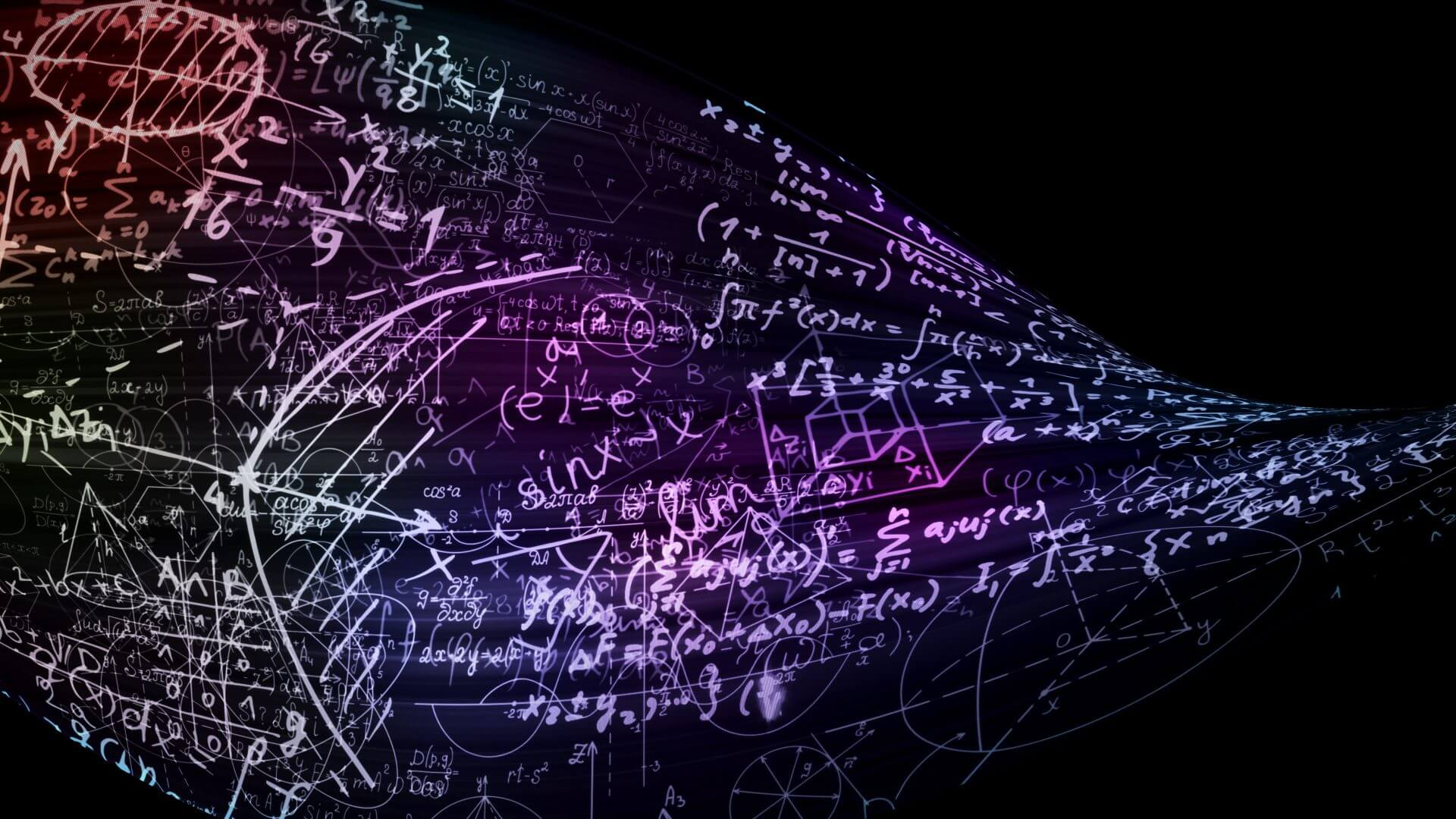April 10, 2023

## Introduction

Calibration is the process of configuring a measurement device such that it provides measurement results within a known and acceptable range. All measurements are susceptible to errors. If these errors can be understood and characterized, they can be mostly removed, resulting in a more accurate answer. It is impossible to remove all errors, as there will always be a certain amount of uncertainty in any measurement. VNA calibration theory helps us understand and statistically quantify this error and state a result in a concise and comprehensive manner.

## Metrology of a Measurement

A metrologically complete measurement is stated as follows:

<Measurement Value> <Units> <Uncertainty Range> <Confidence Interval>

For instance, a measurement of return loss in dB may be:

Return Loss = 12.5 dB ± 0.2 dB with 95% confidence

The true value of the measurement is between 12.3 and 12.7 dB with 95% confidence and there is 5% confidence that the measurement is outside of this range. Metrological principles allow us to calculate the range and confidence interval. There will also be a wider range for which the confidence interval is 98 or 99%, but never 100%.

## Uncertainties

The uncertainty of a measurement is taken to be ± 1σ for a gaussian (normal) distribution value. Since 68% of all possible values lie in this range, the confidence interval is the mean value ±σ with 68% confidence. We may want better confidence, and thus specify a wider interval like ±3σ for 99.7% confidence. This is technically three uncertainties but might still be called the uncertainty. Confusion is eliminated as long as the confidence percentage is clearly stated. For example, the uncertainties on VNA measurements given in Copper Mountain Technologies data sheets are 3σ confidence intervals, as shown in Figure 1 below.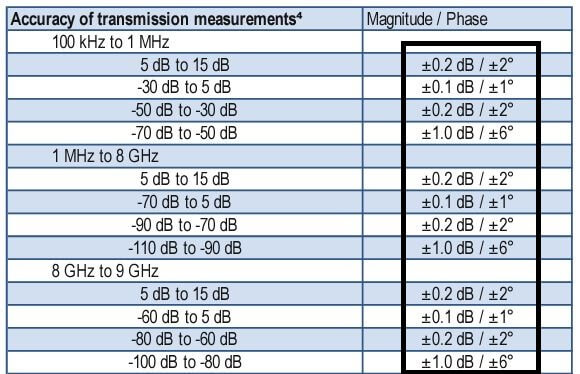Figure 1 – VNA Measurement Uncertainties

Figure 2 below shows a standard (Gaussian) probability distribution function centered at zero with a standard deviation (σ) of 1.0. Probability values are found by integrating the area under the curve between sigma values. The total area under the curve from minus infinity to infinity is 1.0, or 100%, and the area from -1 to +1 sigma is 0.68, or 68%. In practice, the average value –the peak of the curve– may be centered at some non-zero value, and the standard deviation may be a value other than one.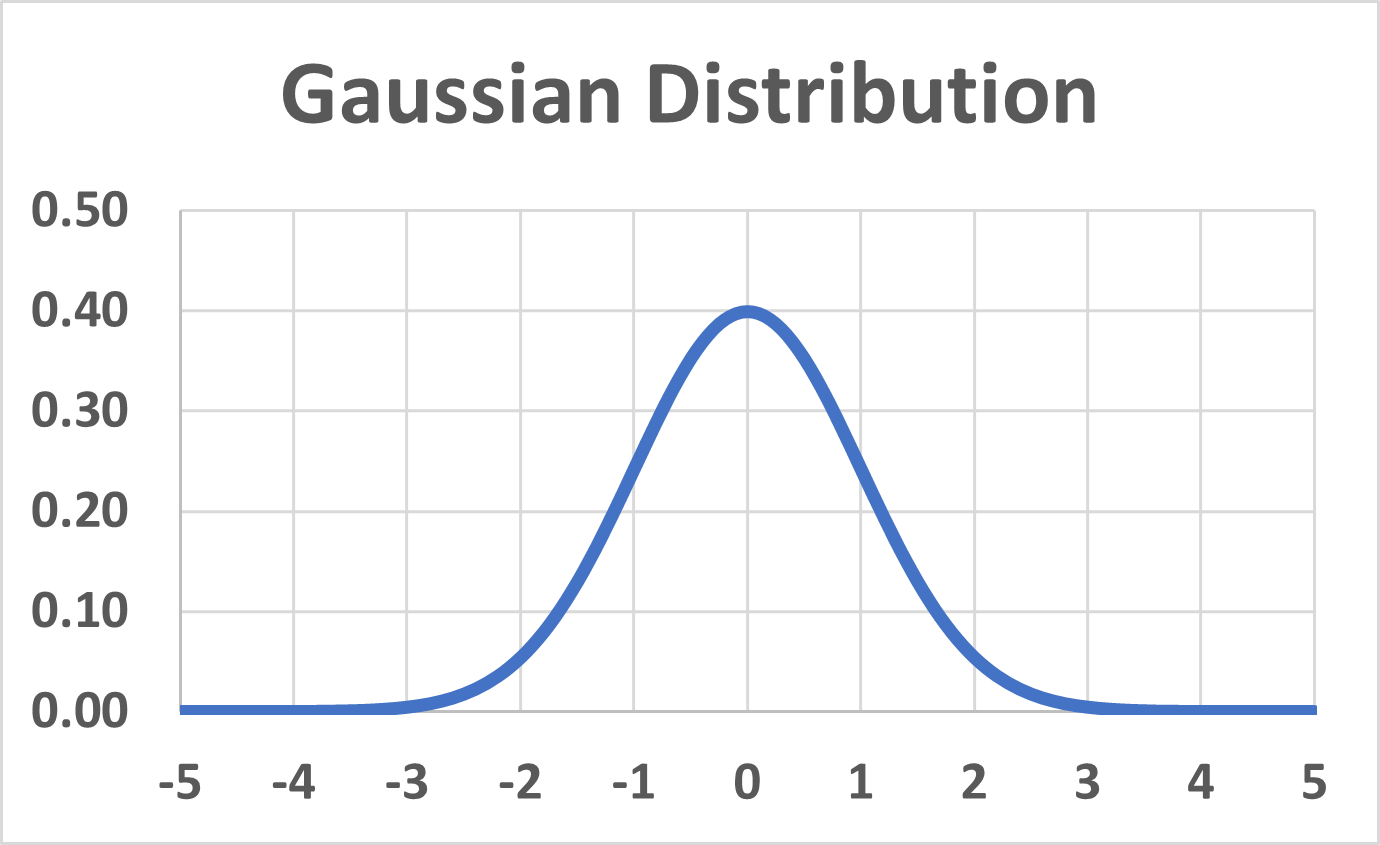Figure 2 – Gaussian Distribution

## VNA Error Terms

An error model assumes there is a Device Under Test (DUT) with error boxes on each side, which are then measured by a perfect VNA. The 12-term error model is favored in the industry, as the terms correlate to real and understandable error sources. This model is shown below in the network flow diagrams of Figure 3 and Figure 4.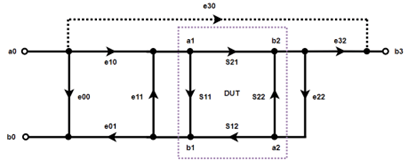Figure 3 – 12 Term Error Model, Forward Direction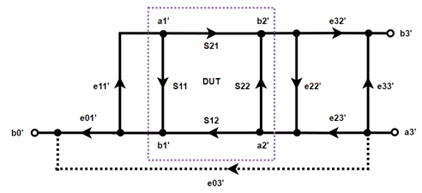Figure 4 – 12 Term Error Model, Reverse Direction

The 12 terms are named as follows:

e00, e33’                      Directivity Error

e10e01, e23’e32           Reflection Tracking Error

e11, e22                      Source Match Error

e10e32, e23’e01           Transmission Tracking Error

e30, e03                      Isolation Error

Network flow diagrams depict waves moving in directions shown by the arrows. The a0 and b0 shown in Figure 3 are not two separate electrical connections. Indeed, they are a single wire. e10 and e01 flow on the same conductor but are separated on the diagram to emphasize the direction of flow. By inspecting the diagram, you can say that signal e11 will add to signal e10 and must flow towards node a1. a0 must be an independent source unaffected by other signals, since no arrows flow toward it. All other nodes must depend on a0 and the S-parameters after it.

The Directivity Error, e00, is due to the leakage in the VNA directional bridge. The two ports on the bridge should produce two pure signals. One, a sample of the incident signal leaving the port, and two, a sample of the reflected signal entering the port. In practice, there is some leakage of the incident signal into the reflection port.

Reflection Tracking, e10e01, accounts for the indignities suffered by an incident signal leaving the port, passing through cables and connectors (e10), and being reflected by the DUT to go through them once again (e01).

Source Match Error, e11, accounts for the complex error in the source impedance of the incident signal as it appears at the input of the DUT. Even if the source impedance of the stimulus of the VNA is a perfect 50 Ohms, small variations in the characteristic impedance of cables and connectors will alter it somewhat.

Transmission Tracking, e10e32, is similar to Reflection Tracking, and includes the first part of it (e10). However, it also includes the errors in the path to the other VNA port, e32.

Load Match Error, e22, is the load impedance error from 50 Ohms as seen at the output of the DUT and includes impedance variations caused by the output cable and connectors and any small load impedance error through the bridge of the VNA itself.

Isolation Error, e30, accounts for any signal which bypasses the DUT entirely. Either through leakage within the VNA itself, which is uncommon, or from the electromagnetic coupling between the two DUT connections, such as that experienced between the probes for a probe station measurement.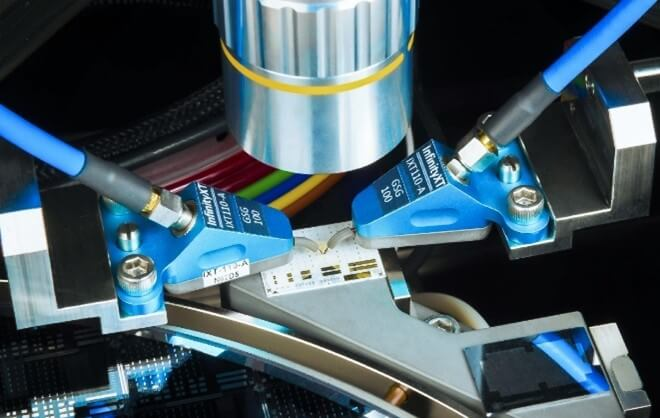Figure 5 – FormFactor Coaxial InfinityXT Probes Measuring ISS Calibration Standards

Normally, the Isolation Error is lower than the VNA receiver noise at even the lowest IF bandwidths and can be safely ignored.

These errors are determined and mostly removed by measuring a series of calibration standards with known characteristics and comparing the actual measurement with error to the expected measurement based on the standard data.

For example, the directivity error is due to a leakage path in the VNA bridge. When a signal is leaving the VNA and there is no reflection, there should be a signal on the forward port of the bridge, but none on the reverse port. Unfortunately, there will always be a small leakage signal from the forward signal, as shown in Figure 6.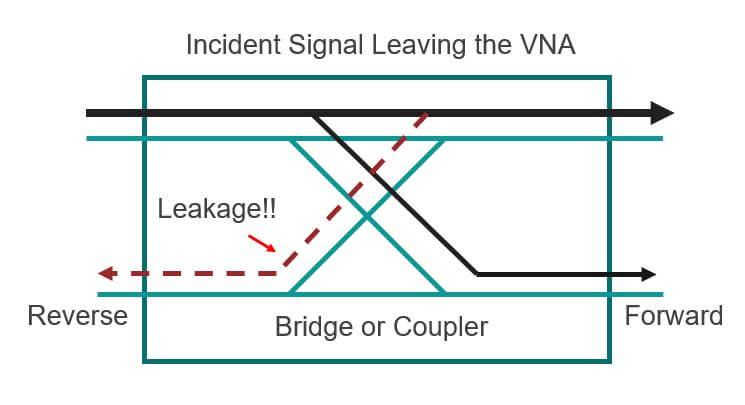Figure 6 – Leakage Signal, Directivity Error

To measure the leakage signal, terminate the port with a perfect load such that there is no real reflection. After the leakage signal is known at all frequencies, it can simply be subtracted from the reflection measurements to remove the directivity error.

Unfortunately, there is no such thing as a perfect load. A \$7,000, 3.5mm, 26.5 GHz calibration load might have a worst-case reflection of -30 dB which would appear on the reflection port of the bridge along with the leakage signal. Therefore, the leakage may only be corrected to this level, and reflection measurements below -30 dB are meaningless. A sliding load calibration will improve on this somewhat but will not be discussed here.

After measuring the calibration standards and mathematically determining all twelve errors, they can be mostly removed from the measurement resulting in a nearly true measurement of the DUT. It is impossible to completely eliminate the errors; they are greatly reduced but not absent. These small remaining errors are called residual errors. For instance, the raw directivity of the VNA bridge might be 18 dB before calibration. After calibration, the residual directivity can be 30 dB to 26.5 GHz with a mechanical calibration kit, or 40 dB using an Automatic Calibration Module (ACM).

## What is SOLT Calibration?

SOLT calibration, or Short-Open-Load-Thru calibration, uses four well-characterized calibration standards. Each of the standards are measured in turn, as shown in Figure 7, and the results are saved in order to compute the measurement correction.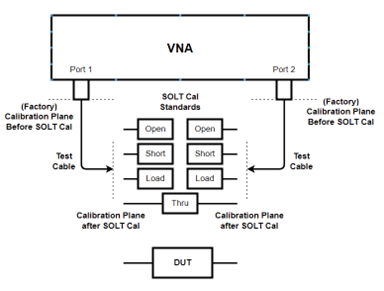Figure 7 – SOLT Calibration

Once all standards are measured—Open-Short-Load on the ends of the test cables and Thru between the cables—the measurements, along with the known values of each standard, are used to calculate all twelve error terms. With the error terms known, a correction matrix can be determined which will mathematically correct all measurements.

The characteristics of the calibration standards are known by one of two methods. First, and most likely, they are defined by a delay between the connector and the actual standard, plus a third order polynomial representing the parasitic effects. The delay and polynomial will be the same for every calibration piece of the same model number from a vendor, so there will be some error due to manufacturing tolerances. Secondly, the calibration kit might have Touchstone data files to characterize each standard . This is much more accurate but requires individual measurement of each standard and is therefore costly.

A typical entry for a calibration kit definition is shown in Figure 8.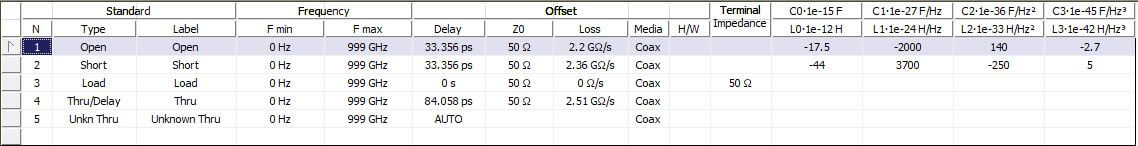Figure 8 – Cal Kit Definition

The delays of each piece are shown and the polynomial coefficients for the parasitic capacitance of the open and the parasitic inductance of the short are on the right-hand side of Figure 8. With this information, the VNA can calculate the true characteristics of each standard.

## What is TRL Calibration?

TRL calibration or the Thru-Reflect-Line method uses transmission lines and shorts or opens for calibration standards . TRL does not require a Load standard, which is the weakest link in the SOLT method. Three standards are employed for TRL calibration three standards:

• a Thru, which can be zero length if the connectors on the ends of the test cables mate directly, or a short length of coaxial line.
• A Reflect standard, which can be any total reflection like a short or open.
• A Line, which is a quarter wavelength longer than the Line at the center frequency of calibration, fc. The Line must be pristine, with precise characteristic impedance and extremely low reflection. Loss in the Line is acceptable. Alternatively, the Line may be data-based if these conditions can’t be met.

TRL is valid over the frequency range where the Line is 20 to 160º longer than the Thru, centered at 90º at fc. If the useable range is f1 to f2 then: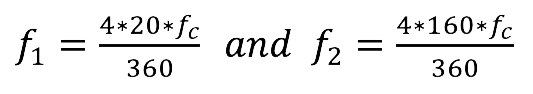For a broader frequency range, multiple Lines can be used for multi-line TRL calibration . TRL will always be band-limited as compared to SOLT, which can go all the way down to zero Hz. Low frequencies require long Lines for TRL, which may not be practical.

The Reflect standard is usually a Short, since it is easiest to implement. A delay between the standard connector and the actual short will result in phase variation over frequency. The phase of the Reflect must be known within ±λ/4 so the delay is usually specified in the TRL calibration kit definition. This requirement comes from the solution of a square root in the correction calculation. The sign of the root is ambiguous without the known approximate phase of the Reflect, and if this sign is chosen incorrectly, subsequent corrected measurements will exhibit an erroneous 180º phase shift.

The best Residual Directivity that can be achieved with SOLT is about 47 dB, due to the uncertainty of the Load standard. However, if a mechanically precise Line with excellent characteristic impedance is used for TRL, this can be improved to as high as 60 dB, allowing accurate VNA reflection measurements to much lower levels.

TRL correction comes from the solution of an 8-term error correction model which does not correlate directly with physical sources of errors. The network flow diagram is shown below in Figure 9.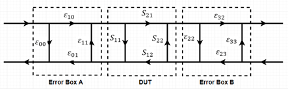Figure 9 – 8-Term Calibration Model

The process of correction amounts to solving for all eight error terms, converting to Cascade Parameters which may be multiplied directly (unlike S-parameters), solving for the inverse, and multiplying left and right sides to leave only the DUT.

Starting with measured S parameters with error, Sm:

Sm = [Sa] [SDUT] [Sb]

Convert to equivalent cascade parameter measurement Tm: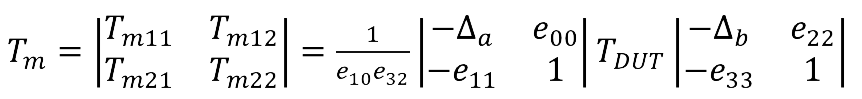Where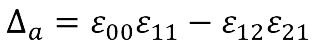and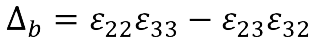(the determinants)

Tm is measured, TDUT is unknown. Then: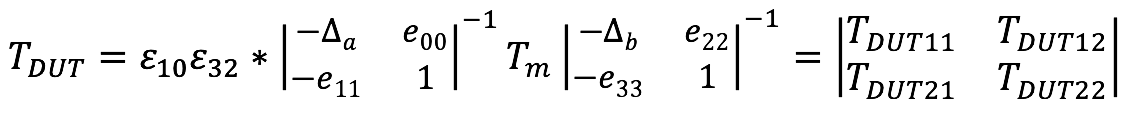Convert the cascade parameters back to S-ParametersWhere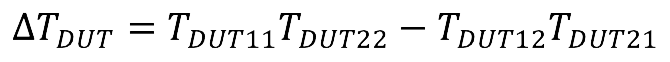And you have the corrected result. The process of finding the eight error terms is beyond the scope of this application note but is explored in .

## Conclusion:

To achieve accurate results, calibrate the VNA using a defined calibration kit prior to making a measurement. The two most common methods, SOLT and TRL, were explained here. This user calibration should be differentiated from factory calibration. For a factory calibration, the VNA is examined at a certified test laboratory and verified to be performing within acceptable operating parameters. The ISO-17025 certified laboratory at Copper Mountain Technologies is capable of performing this service. It is common to have factory calibration performed every two years, but different organizations may have different required calibration intervals.